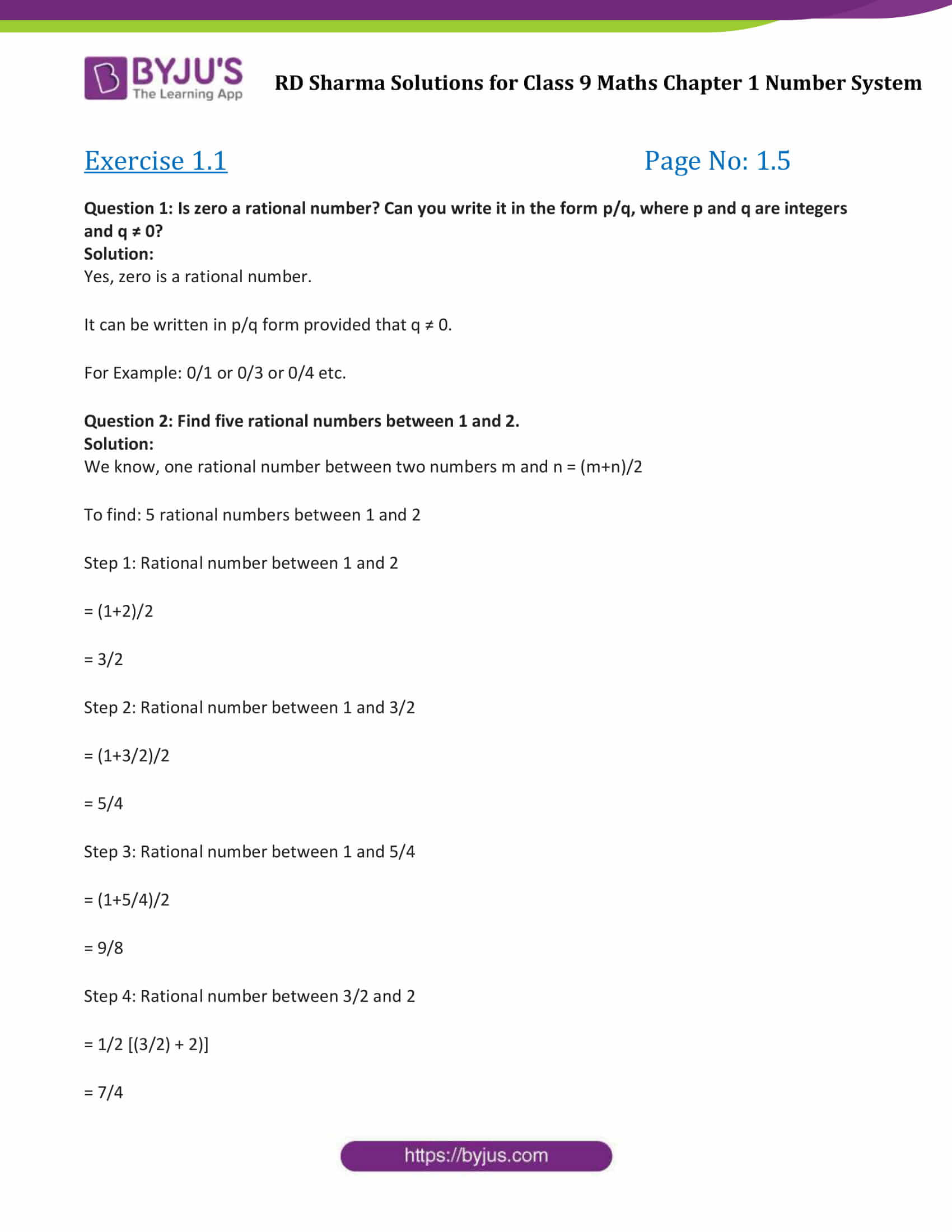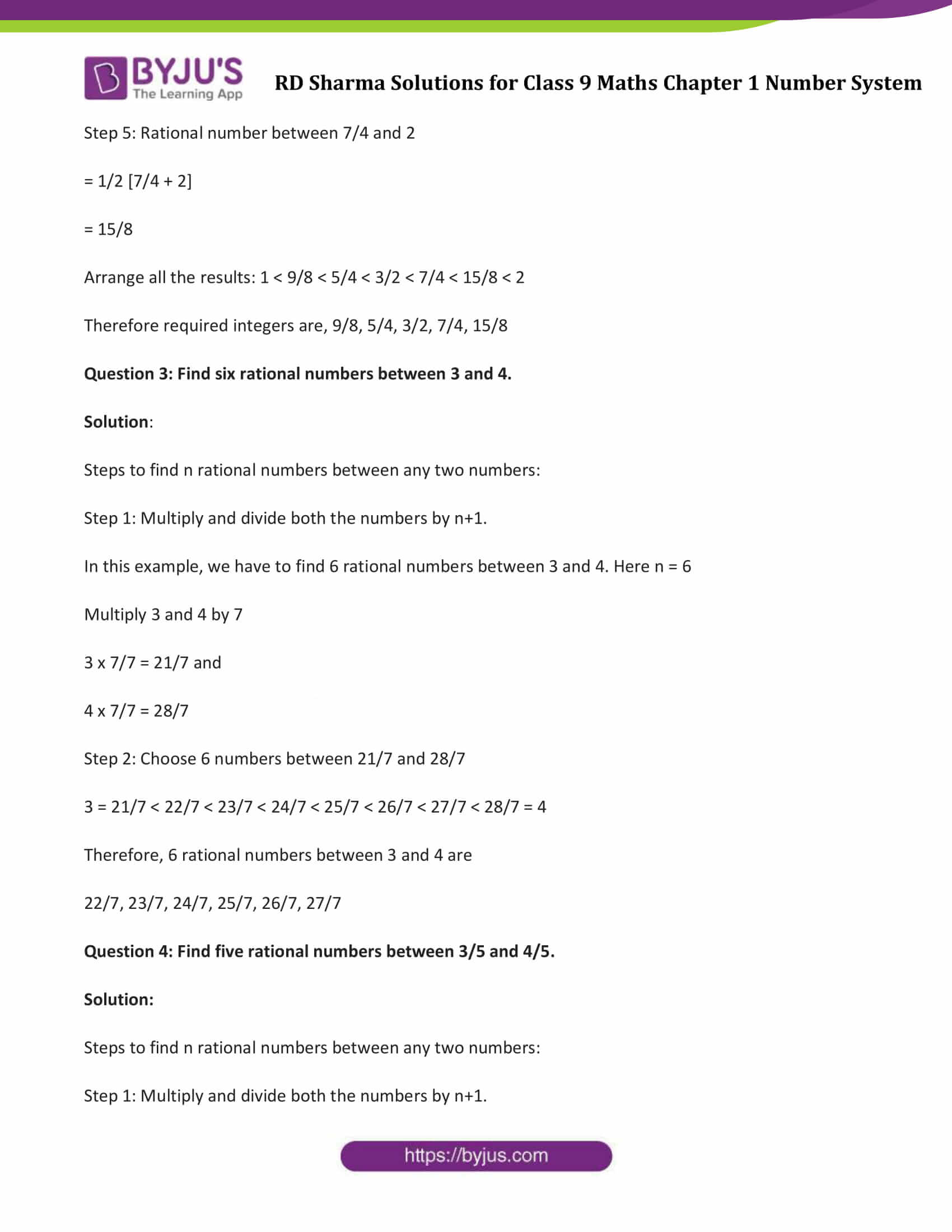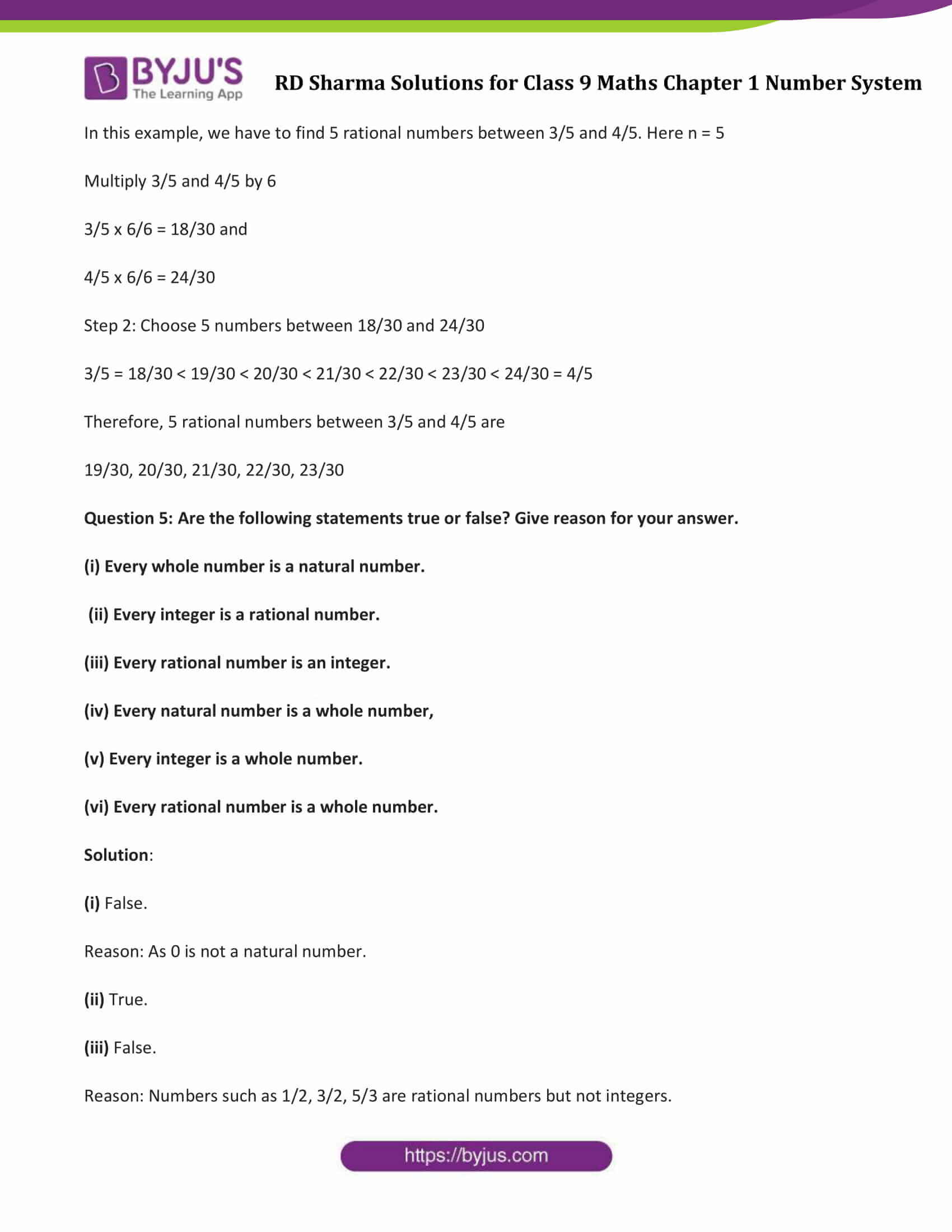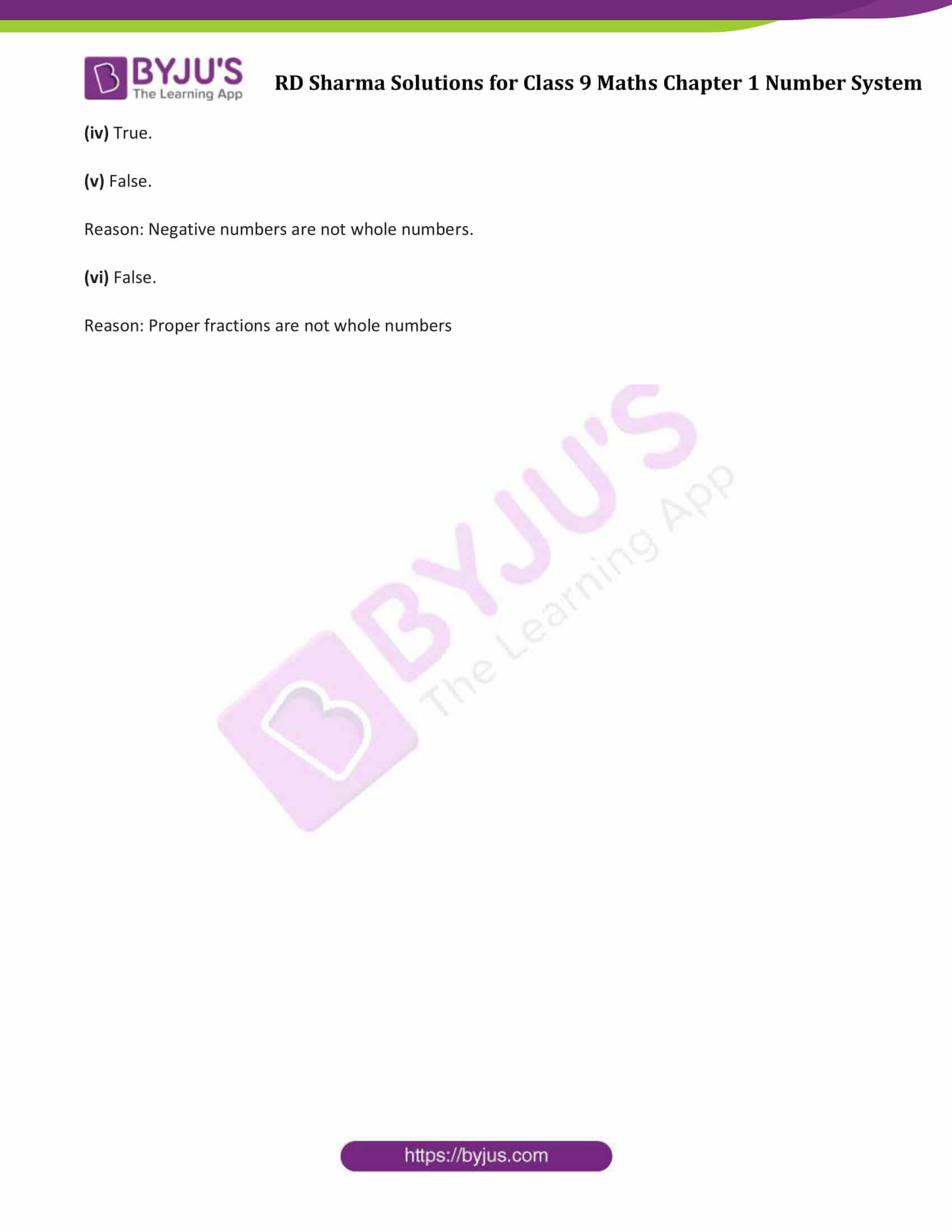# RD Sharma Solutions for Class 9 Maths Chapter 1 Number System Exercise 1.1

RD Sharma Solutions for Class 9 Maths Chapter 1 exercise 1.1 is available here. All problems of RD Sharma chapter 1 are prepared by subject experts to help students clear their doubts. This exercise is based on the basic concepts of whole numbers, natural numbers, integers, rational and irrational numbers. Students can download Maths Chapter 1 Class 9 RD Sharma Solutions and start practicing offline.

## Download PDF of RD Sharma Solutions for Class 9 Maths Chapter 1 Number System Exercise 1.1### Access Answers to Maths RD Sharma Solutions for Class 9 Chapter 1 Number System Exercise 1.1 Page number 1.5

Question 1: Is zero a rational number? Can you write it in the form p/q, where p and q are integers and q ≠ 0?
Solution:

Yes, zero is a rational number.

It can be written in p/q form provided that q ≠ 0.

For Example: 0/1 or 0/3 or 0/4 etc.

Question 2: Find five rational numbers between 1 and 2.
Solution:

We know, one rational number between two numbers m and n = (m+n)/2

To find: 5 rational numbers between 1 and 2

Step 1: Rational number between 1 and 2

= (1+2)/2

= 3/2

Step 2: Rational number between 1 and 3/2

= (1+3/2)/2

= 5/4

Step 3: Rational number between 1 and 5/4

= (1+5/4)/2

= 9/8

Step 4: Rational number between 3/2 and 2

= 1/2 [(3/2) + 2)]

= 7/4

Step 5: Rational number between 7/4 and 2

= 1/2 [7/4 + 2]

= 15/8

Arrange all the results: 1 < 9/8 < 5/4 < 3/2 < 7/4 < 15/8 < 2

Therefore required integers are, 9/8, 5/4, 3/2, 7/4, 15/8

Question 3: Find six rational numbers between 3 and 4.

Solution:

Steps to find n rational numbers between any two numbers:

Step 1: Multiply and divide both the numbers by n+1.

In this example, we have to find 6 rational numbers between 3 and 4. Here n = 6

Multiply 3 and 4 by 7

3 x 7/7 = 21/7 and

4 x 7/7 = 28/7

Step 2: Choose 6 numbers between 21/7 and 28/7

3 = 21/7 < 22/7 < 23/7 < 24/7 < 25/7 < 26/7 < 27/7 < 28/7 = 4

Therefore, 6 rational numbers between 3 and 4 are

22/7, 23/7, 24/7, 25/7, 26/7, 27/7

Question 4: Find five rational numbers between 3/5 and 4/5.

Solution:

Steps to find n rational numbers between any two numbers:

Step 1: Multiply and divide both the numbers by n+1.

In this example, we have to find 5 rational numbers between 3/5 and 4/5. Here n = 5

Multiply 3/5 and 4/5 by 6

3/5 x 6/6 = 18/30 and

4/5 x 6/6 = 24/30

Step 2: Choose 5 numbers between 18/30 and 24/30

3/5 = 18/30 < 19/30 < 20/30 < 21/30 < 22/30 < 23/30 < 24/30 = 4/5

Therefore, 5 rational numbers between 3/5 and 4/5 are

19/30, 20/30, 21/30, 22/30, 23/30

Question 5: Are the following statements true or false? Give reason for your answer.

(i) Every whole number is a natural number.

(ii) Every integer is a rational number.

(iii) Every rational number is an integer.

(iv) Every natural number is a whole number,

(v) Every integer is a whole number.

(vi) Every rational number is a whole number.

Solution:

(i) False.

Reason: As 0 is not a natural number.

(ii) True.

(iii) False.

Reason: Numbers such as 1/2, 3/2, 5/3 are rational numbers but not integers.

(iv) True.

(v) False.

Reason: Negative numbers are not whole numbers.

(vi) False.

Reason: Proper fractions are not whole numbers

## RD Sharma Solutions for Class 9 Maths Chapter 1 Number System Exercise 1.1

RD Sharma Solutions Class 9 Maths Chapter 1 Number System Exercise 1.1 is based on the following topics and subtopics:

• Number System Introduction
• Review of Numbers
• Whole numbers, natural numbers and integers
• Rational and irrational numbers